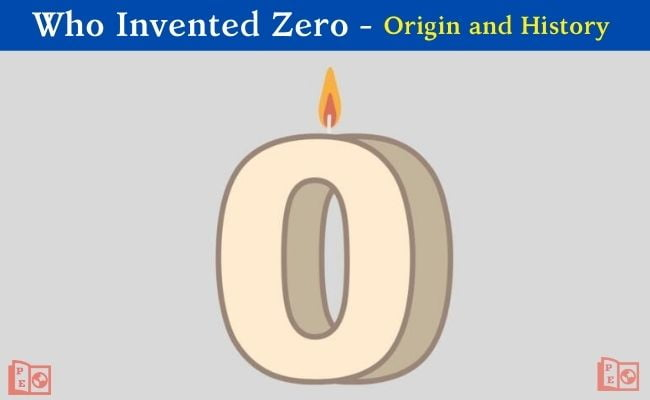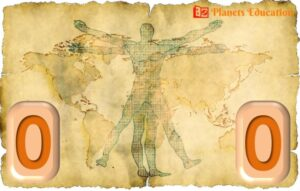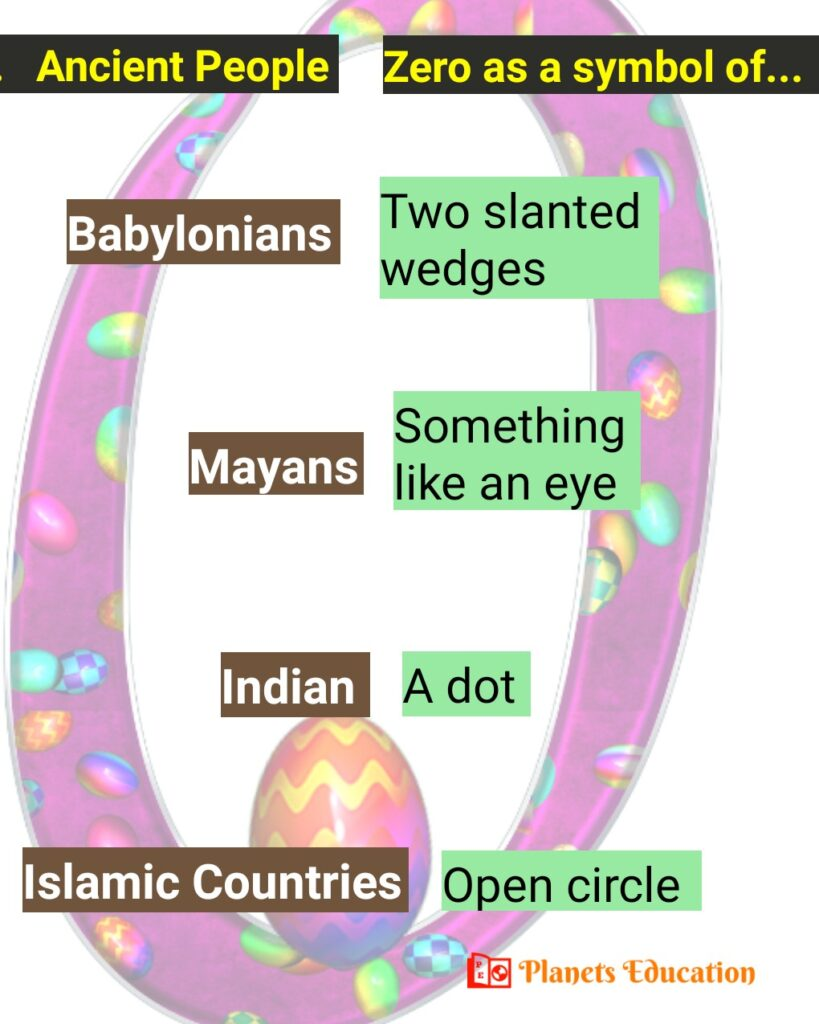# Who Invented Zero, Do You Really Know!Zero 0 is a number and digit that represents the concept of ‘nothing’ or the absence of any quantity. It is believed that ancient people were understanding the meaning of nothing but they had no proper concept or symbol to use in mathematics before the 5th century.

The Zero in mathematics and in our daily life is as important as other digits. Here in this article, we have mentioned: “who invented zero or who discovered zero, history of zero, and other important topics regarding the invention of the zero”.

For the invention of zero, most credit goes to 2 Indian mathematicians and astronomers Aryabhatta and Brahmagupta. Though Sumerians in Mesopotamia civilization, Babylonians, and Meso-americans also used zero as a symbol.

The proper mathematical uses of zero started in the 7th century by Brahmagupta. He denoted it as a placeholder with a symbol of a dot under the numbers. “Zero not only represents nothing but it also represents the starting point of anything”.

#### Name of Zero

The name for the number ‘0’ in English is ‘zero’ which is derived from the Italian word ‘zefiro’. It is called ‘shunya’ in India in the Hindi language and ‘sifr’ in the middle east in the Arabic language. There are many more words that represent zero, such as “nothing, naught, nil, none”.

## Who invented Zero: History

There was no symbol for zero around 800 AD ago. Firstly, it came into existence independently as a symbol in the Hindu-Arabic number system with a base of 10. In ancient times many civilizations used the number system for counting.#### Zero in Mesopotamian Civilization

It is said that the Sumerians were the first people in the world who develop a counting system around 4000 years ago. Babylonians got their number system from the Sumerians with a base of 60.

Babylonians left an empty space for representing zero in their number system but it was so confusing in larger numerals. At around 300 BC they started using zero as a symbol of ‘two slanted wedges’ in larger numerals but they never developed the idea for zero as a number independently.

#### Zero in Maya (Mesoamerican) Civilization

The Mayans (south-central Mexico and Central America) used the concept of zero in their long count calendar. The number system of the Mayans had a base of 20 and they use it in the calendar as a symbol of something like an eye. They never used zero in equations or independently in numbers.

#### Zero in India

The main credit goes to the Indians to use zero independently. Indians called it ‘shunya’ in the Sanskrit language which represents the meaning of “empty/ void/ space”. They used it in their Shlokas (poems). The Indian mathematician and astronomer Aryabhatta (476-550 AD) utilize the concept of zero independently in his mathematical works but he did not use it as a symbol.

Another Indian mathematician Brahmagupta (598-668 AD) use the zero in their mathematical equations with its operations and rules. At the time Brahmagupta including other Indians used the zero as a placeholder. They used it under the numbers with a symbol of a “dot” and considered the meaning of this dot as null.

Brahmagupta was the first person who describes a mathematical rule “if a number is subtracted by the same number, the result will be zero.”

Brahmagupata gave many other mathematical rules, like:

• The Sum of two positive numbers would be equal to a positive number, e.g. 5+3=8.
• Sum of two negative numbers would be equal to a negative number, e.g. (-3)+(-2)=(-5)
• The Sum of a positive and a negative would be equal to their difference, if both are equal it would be zero, e.g. 5+(-3)=2
• The Sum of negative and zero would be equal to a negative, e.g. (-3)+0= -3
• Sum of positive and zero would be equal to a positive, e.g. 5+0= 5

Brahmagupta also describes the operations on negative numbers. Indians were using zero as a large dot symbol.

#### Zero in Islamic Countries, China, and Europe

Zero spread in Islamic countries and in China at the end of the 8th century. In about 825 AD, a Persian mathematician Musa-Al-Khwarizmi use the little circle as a symbol of zero in his mathematical operations.Origin and History of Zero

The Hindu-Arabic numeral system reached Europe at about the end of the 11th century. Some religious leaders oppose the use of zero in Europe because of their belief in ‘nothingness means devil’. But the European merchants used it illegally and by the end of the 13th century some books got published for mathematical operations, so it became common in Europe also.

## Zero as a placeholder

Now with the help of ‘1…9’ digits and ‘0’, we can represent any numerals. The most common number system we use is the Hindi-Arabic number system. There are ten digits “0, 1, 2, 3, 4, 5, 6, 7, 8, 9” and the group of digits is called ‘numerals’. In the numerals, we can use zero as a placeholder in the ‘place value system’. For example, for numeral 4356 the place value of ‘3’ is 300.Binary Number ‘0 and 1’

Now zero is being widely used in a binary numeral system with base 2. It uses only two digits ‘0’ and ‘1’. In digital electronics like a computer, this number system is being used.

## Invention or Discovery of Concept of Zero

Well, in a meaningful term if we talk about “who invented zero” then this question itself is not right. The right question would be “discovery of zero” or “who discovered zero first”. It is because there is a lot of difference between the discovery and invention of the term. And giving someone credit for this would be inappropriate.

So the real thing is Zero was Discovered not invented. Either we can say the concept of zero was discovered.

Discovery is to know something that already exists, and the invention is to create something totally new.  Zero was already present and some mathematicians just discovered the concept of it. They gave the rules to compute with it.

## Conclusion for ‘Who Invented Zero’?

If we conclude “who invented zero first“, then we can usually say many gave their contribution. Most civilizations were using it in ancient times as a symbol. But the first use of it as a place holder did by the Indians. And, here today we use zero as an oval shape in our number system.

Check Also: